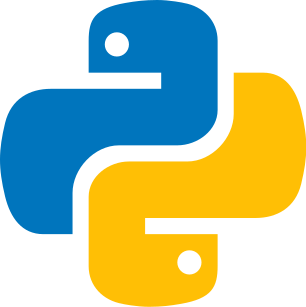Codistwa

# Learn Recursion / recursive functions

## in Programming

Last update : February 11th, 2022

Recursion means that a function calls itself. This is done by running the internal program with the variable decreasing until a limit is reached. The purpose is to perform loops with an elegant syntax. The disadvantage is that the execution of this type of function is very slow. Indeed, the complexity is exponential. So you have to be careful to place a condition at the beginning of the function body to avoid an infinite execution that could crash the program.### Recursion with one parameter

As a reminder, the factorial corresponds to this calculation: 3! = 1 × 2 × 3 = 6. It is the product of strictly positive integers less than or equal to n characterized by an exclamation mark.

The calculation of the factorial is done as follows: n! = n * (n-1) * (n-2) * (n-3) * .... 1 with 1! = 1.

The recursive version of the factorial is: n! = n * (n-1)!

Thus, computing the factorial n is the same as computing the product of n by the factorial (n-1). This is what we will implement here.

When n reaches 1, the code stops because we don't need to calculate the sequence anymore. After all, an element multiplied by 1 is equal to itself.

#### Pass an exam

The character must repeat the same steps (reading the teacher's lectures, doing the exercises) until he/she is ready for his/her exam. The goal (the base) will correspond to obtaining a good grade. So as long as the grade is not high, i.e., an A+, you have to continue the cycle: revise (read the courses + do the exercises). So these are small tasks, sub-problems.

#### Codepython
1def factorial(n):
2    if n == 1:
3        return n
4    else:
5        return n * factorial(n - 1)
6
7print(factorial(5)) # 120

Time complexity : factorial

Before

5

Result

120

### Recursion with two parameters

With two parameters we will be able to calculate the power of a number.

Thus, 2³ = 8 because 2 x 2 x 2 = 8. As you can see we are still calculating a series.

As usual we put a condition to avoid an infinite execution, so if the exponent is equal to zero we return 1 because 2⁰ = 1.

#### Codepython
1def power(base, exponent):
2    if exponent == 0:
3        return 1
4    return base * power(base, exponent - 1)
5
6print(power(2, 3))  # 8
7

Time complexity : exponential

#### FormulaA recursive function is a function that calls itself. It allows performing loops elegantly to calculate sequences. However, you will have to be careful with the data because the execution of this function is very slow due to its exponential complexity.